#### Chapter 17 Construction R.D. Sharma Solutions for Class 9th Exercise 17.3

Exercise 17.3

1Construct a Δ ABC in which BC = 3.6 cm, AB + AC = 4.8 cm and ∠B = 60°.

Solution

We are asked to construct a triangle ABC in which BC = 3.6 cm, ∠B = 60° and AB + AC = 4.8 cm
We will follow the following algorithm for the construction .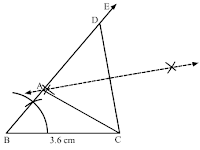Steps of construction

Step 1: Draw BC of 3.6 cm
Step 2: Draw ∠EBC = 60°.
Step 3: From ray BE, cut BD of 4.8 cm.
Step 4: Draw segment DC.
Step 5: Draw the perpendicular bisector of DC, intersecting the ray BE at A
Step 6: Draw segment AC.

ΔABC is the required angle.

2. Construct a Δ ABC in which AB + AC = 5.6 cm, BC = 4.5 cm and ∠B = 45°.

Solution

We are asked to construct a triangle ABC in which BC = 4.5 cm and AB + AC = 5.6 cm .
We will follow the following algorithm for the construction .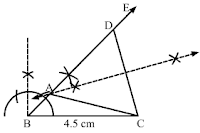Steps of construction

Step 1: Draw BC of 4.5 cm.
Step 2: Draw ∠EBC = 45°
Step 3: From ray BE, cut BD of 5.6 cm.
Step 4: Draw segment DC.
Step 5: Draw the perpendicular bisector of DC, intersecting the ray BE at A
Step 6: Draw segment AC.

ΔABC is the required angle.

3. Construct a Δ ABC in which BC = 3.4 cm, AB − AC = 1.5 cm and ∠B = 45°.

Solution

We are asked to construct a triangle ABC in which BC = 3.4 cm , and ∠B = 45° and AB - AC = 1.5 cm
We will follow the following algorithm for the construction .

Steps of construction

Step 1: Draw BC of 3.4 cm.
Step 2: Draw ∠CBE = 45°
Step 3: From the ray BE, cut BD of 1.5 cm.
Step 4: Draw segment DC.
Step 5: Draw the perpendicular bisector of DC, intersecting the ray BE at A
Step 6: Draw AC.

ΔABC is the required angle.

4. Using ruler and compasses only, construct a ΔABC, given base BC = 7 cm, ∠ABC = 60° and AB+AC = 12 cm.

Solution

We are asked to construct a triangle ABC in which BC = 7 cm, ∠B = 60° and AB + AC = 12 cm
We will follow the following algorithm for the construction .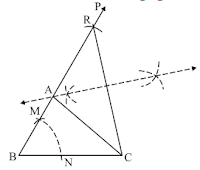Steps of construction

Step 1: Draw BC of length 7 cm.
Step 2: With B as a centre, and convenient radius draw an arc cutting BC at N.
Step 3: With N as a centre, and same radius draw an arc cutting the previous arc at M.
Step 4: Draw BM and produce it to P.
Step 5: From BP, cut BR = 12 cm.
Step 6: Draw segment RC.
Step 7: Draw perpendicular bisector of RC. It cuts the ray BR at A.
Step 8: Draw AC.
ΔABC is the required triangle.

5. Construct a triangle whose perimeter is 6.4 cm, and angles at the base are 60° and 45°.

Solution

We are asked to draw triangle ABC whose base angles are 60° and 45° and its perimeter is 6.4 cm
We will follow the following algorithm for the construction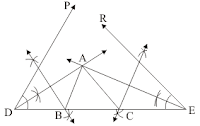Steps of construction

Step 1: Draw DE of 6.4 cm.
Step 2: Draw ∠EDP =∠B = 60° and ∠DER = ∠C = 45°.
Step 3: Draw the angle bisectors of ∠EDP and ∠DER and mark their point of intersection as A.
Step 4: Draw the perpendicular bisectors of AD and AE. They meet DE at point B and Point C respectively.
Step 5: Draw AB and AC to get the required ΔABC.

6. Using ruler and compasses only, construct a Δ ABC from the following data:
AB + BC + CA = 12 cm, ∠B = 45° and ∠C = 60°.

Solution

We are asked to draw triangle ABC whose base angles are 60° and 45° and its perimeter is 12 cm
We will follow the following algorithm for the construction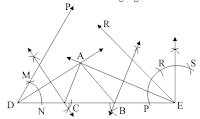Steps of construction

Step 1: Draw DE of length 12 cm.
Step 2: Construct ∠EDP =∠C = 60° and ∠DER =∠B = 45°, using regular methods.
Step 3: Draw the angle bisectors of ∠EDP and ∠DER and mark their point of intersection as A.
Step 4: Draw the perpendicular bisectors of AE and AD. They meet DE at point B and Point C respectively.
Step 5: Draw AB and AC to get the required ΔABC.

7. Construct a right-angled triangle whose perimeter is equal to 10 cm and one acute angle equal to 60°.

Solution

We are asked to draw triangle ABC whose base angles are 60° and 90° and its perimeter is 10 cm.
We will follow the following algorithm for the constructionSteps of construction

Step 1: Draw a line segment DE of 10 cm.
Step 2: Draw ∠EDM = ∠B = 90° and ∠DEN = ∠C = 60°.
Step 3: Draw the angle bisectors of ∠EDM and ∠DEN and mark their point of intersection as A.
Step 4: Draw the perpendicular bisectors of AD and AE. They meet DE at B and C respectively.
Step 5: Draw AB and AC to get the desired ΔABC.

8. Construct a triangle ABC such that BC = 6 cm, AB = 6 cm and median AD = 4 cm.

Solution

We are given BC = 6 cm, and median AD = 4 cm
We have to construct the triangle ABC
We will follow the following algorithm for the construction .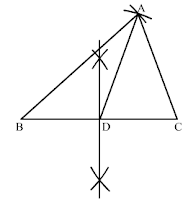Steps of construction

Step 1: Draw a line segment BC of length 6 cm.
Step 2: Draw the perpendicular bisector of line BC cutting the line at point D, such that BD = 3 cm.
Step 3: With centre B and radius = 6 cm, draw an arc.
Step 4: With centre D and radius = 4 cm, draw an arc intersecting the previous arc at A.
Step 5: Draw AC to get the desired ΔABC.

9. Construct a right triangle ABC whose base BC is 6 cm and the sum of hypotenuse AC and other side AB is 10 cm.

Solution

We have to construct a right triangle ABC with base BC = 6 cm and the sum of hypotenuse and the other side is 10 cm
We will follow the following algorithm for the construction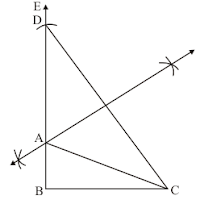Steps of construction

Step 1: Draw a line segment BC of length 6 cm.
Step 2: Draw ∠CBE = 90°
Step 3: From BE, cut BD of length 10 cm.
Step 4: Draw the line segment DC.
Step 5: Draw the perpendicular bisector of DC, intersecting the ray BE at point A
Step 6: Draw AC to get the desired ΔABC.

10. Construct a triangle XYZ in which ∠Y = 30°, ∠Z = 90° and XY + YZ + ZX = 11.

Solution

We are asked to construct a triangle XYZ such that ∠Y = 30°, ∠Z = 90° XY + YZ + ZX = 11 cm
We will follow the following algorithm for the constructionSteps of construction-

Step 1: Draw a line segment AB of length 11 cm.
Step 2: Draw ∠BAC = ∠Y = 30 and ∠ABD = ∠Z = 90°
Step 3: Bisect ∠BAC and ∠ABD and mark the point of intersection of these angle bisectors by X.
Step 4: Draw perpendicular bisectors of AX and XB. They meet AB at point Y and Z, respectively.
Step 5: Draw XY and XZ to get the desired ΔXYZ.